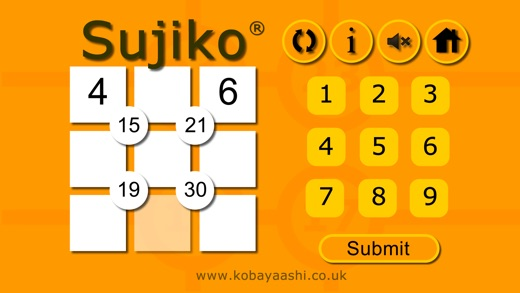## Friday, December 15, 2017

### Sujiko (English) by Kobayaashi Studios \$.99 and Sujiko2015 FREESujiko is a tough little puzzle. Assume that the top space is A. The middle row is labeled B, C & D

First equation: A + B + C + 4 =15
Second equation: A + C + D + 6 = 21

From those we get that D - B = 4.  There are only 2 possibilities: B = 1 while D = 5   and B = 3 while D = 7.  We plug them in and go from there.

I don't think the image above is solve-able. The 30 should be surrounded by 6, 7, 8 & 9, and 6 is already used.

Below are the rules and the links to 2 versions of this game, made by the same people.

The playboard is composed of a 3 x 3 grid, with four circular spaces at the internal intersecting nodes of the grid. The objective is to "Place the numbers 1 to 9 in the spaces so that the number in each circle is equal to the sum of the four surrounding spaces." At least one number in the grid is filled in on most examples to ensure that each puzzle has only one possible solution.

https://itunes.apple.com/us/app/sujiko-english/id1319373995?mt=8&ign-mpt=uo%3D2  \$.99

https://itunes.apple.com/us/app/sujiko2015/id1027053814  FREE